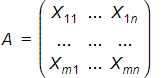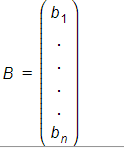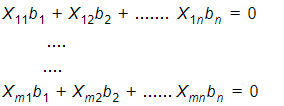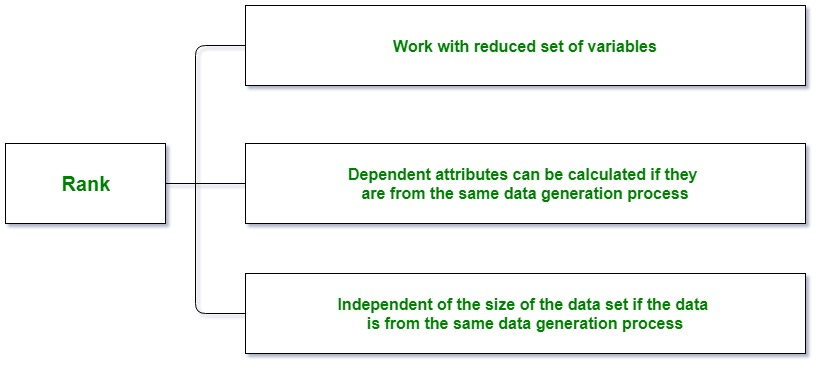# Null Space and Nullity of a Matrix

• Difficulty Level : Hard
• Last Updated : 18 May, 2022

Null Space and Nullity are concepts in linear algebra which are used to identify the linear relationship among attributes.

### Null Space:

The null space of any matrix A consists of all the vectors B such that AB = 0 and B is not zero. It can also be thought as the solution obtained from AB = 0 where A is known matrix of size m x n and B is matrix to be found of size n x k. The size of the null space of the matrix provides us with the number of linear relations among attributes.A generalized description:

Let a matrix beand there is one vector in the null space of A, i.e,then B satisfies the given equations,The idea –

1. AB = 0 implies every row of A when multiplied by B goes to zero.
2. Variable values in each sample(represented by a row) behave the same.
3. This helps in identifying the linear relationships in the attributes.
4. Every null space vector corresponds to one linear relationship.

### Nullity:

Nullity can be defined as the number of vectors present in the null space of a given matrix. In other words, the dimension of the null space of the matrix A is called the nullity of A. The number of linear relations among the attributes is given by the size of the null space. The null space vectors B can be used to identify these linear relationship.

Rank Nullity Theorem:
The rank-nullity theorem helps us to relate the nullity of the data matrix to the rank and the number of attributes in the data. The rank-nullity theorem is given by –

Nullity of A + Rank of A = Total number of attributes of A (i.e. total number of columns in A)

Rank:
Rank of a matrix refers to the number of linearly independent rows or columns of the matrix.Example with proof of rank-nullity theorem:

Consider the matrix A with attributes {X1, X2, X3}
1  2  0
A = 2  4  0
3  6  1
then,
Number of columns in A = 3R1 and R3 are linearly independent.
The rank of the matrix A which is the
number of non-zero rows in its echelon form are 2.
we have,
AB = 0Then we get,
b1 + 2*b2 = 0
b3 = 0
The null vector we can get isThe number of parameter in the general solution is the dimension
of the null space (which is 1 in this example). Thus, the sum of
the rank and the nullity of A  is 2 + 1 which
is equal to the number of columns of A.


This rank and nullity relationship holds true for any matrix.

Python Example to find null space of a Matrix:

 # Sympy is a library in python for # symbolic Mathematicsfrom sympy import Matrix  # List A A = [[1, 2, 0], [2, 4, 0], [3, 6, 1]]  # Matrix AA = Matrix(A)  # Null Space of ANullSpace = A.nullspace()   # Here NullSpace is a list  NullSpace = Matrix(NullSpace)   # Here NullSpace is a Matrixprint("Null Space : ", NullSpace)  # checking whether NullSpace satisfies the# given condition or not as A * NullSpace = 0# if NullSpace is null space of Aprint(A * NullSpace)

Output:

Null Space :  Matrix([[-2], , ])
Matrix([, , ])


Python Example to find nullity of a Matrix:

 from sympy import Matrix  A = [[1, 2, 0], [2, 4, 0], [3, 6, 1]]   A = Matrix(A)  # Number of ColumnsNoC = A.shape  # Rank of Arank = A.rank()  # Nullity of the Matrixnullity = NoC - rank  print("Nullity : ", nullity)

Output:

Nullity :  1


My Personal Notes arrow_drop_up